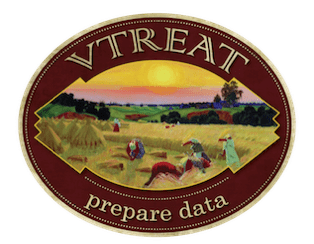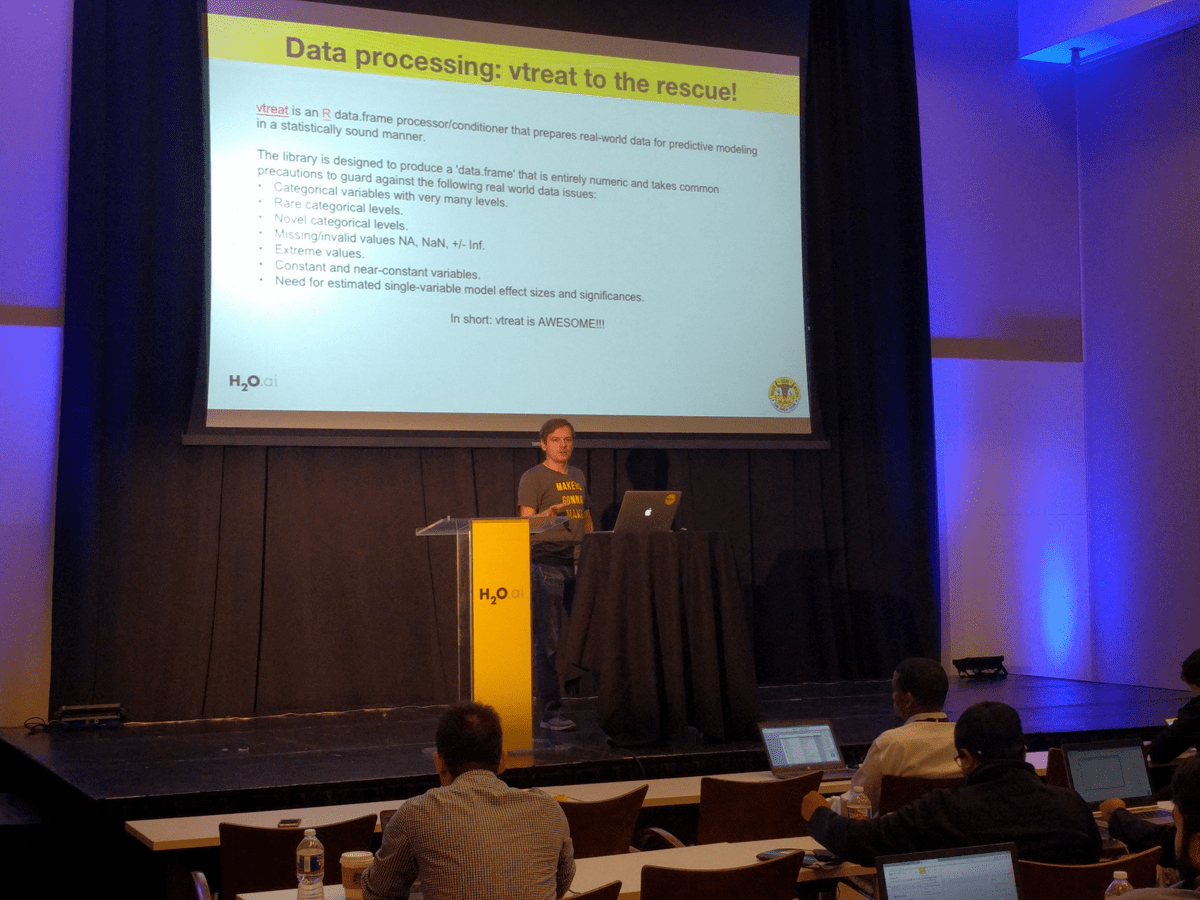# vtreat: prepare data

This article is on preparing data for modeling in `R` using `vtreat`.## Our example

Suppose we wish to work with some data. Our example task is to train a classification model for credit approval using the `ranger` implementation of the random forests method. We will take our data from John Ross Quinlan's re-processed "credit approval" dataset hosted at Lichman, M. (2013). UCI Machine Learning Repository, http://archive.ics.uci.edu/ml; Irvine, CA: University of California, School of Information and Computer Science.

For convenience we have copied the data to our working directory here. We start by loading the data, identifying the outcome, and splitting the data into training and evaluation portions:

```# load data
'crx.data.txt',
sep = ',',
stringsAsFactors = FALSE,
na.strings = '?'
)

# prepare outcome column and level
outcome <- 'V16'
positive <- '+'
d[[outcome]] <- as.factor(d[[outcome]])

# identify variables
vars <- setdiff(colnames(d), outcome)

# split into train and test/evaluation
set.seed(25325)
isTrain <- runif(nrow(d)) <= 0.8
dTrain <- d[isTrain, , drop = FALSE]
dTest <- d[!isTrain, , drop = FALSE]
rm(list = 'd')
```

## Without `vtreat`

We could try to model directly on the original variables without `vtreat` as below.

```library("ranger")
f <- paste(outcome,
paste(vars, collapse = ' + '),
sep = ' ~ ')
model <- ranger(as.formula(f),
probability = TRUE,
data = dTrain)
#  Error: Missing data in columns: V1, V2, V4, V5, V6, V7, V14.
```

`ranger` signaled it did not want to work with this data as there are variables missing values in the training set. This is only one of the many potentially analysis ruining issues that can be lurking in real world data (and as always, detected or signaled errors are better news than undetected errors!).

We name just a few of the issues that could be lurking in real world data:

– Missing values.
– Categorical variable levels that occur in the evaluation set, but were not in the training set (bad luck).
– Categorical variables with large sets of levels.

## With `vtreat`

`vtreat` is designed to prepare your data for analysis in a statistically sound manner. After `vtreat` processing all columns are numeric, there are no missing values, and the information in the original data is preserved (modulo user discretion in selecting variables and categorical variable levels).

`vtreat::prepare()` is roughly a powered-up replacement for `stats::model.matrix()` (which itself is implicit in many `R` modeling work-flows).

Let's finish the modeling task with `vtreat`.

### Cross frame experiment

First we run a "cross frame experiment." A cross frame experiment is a sophisticated modeling step that:

– Collects statistics on the relations between your original modeling variables and the training outcome.
– Introduces proposed new transformed modeling variables.
– Produces a "simulated out of sample" training frame that is a version of your model training data prepared in such a way as to simulate having been produced without having looked at that same data during the design of the variable transformations. This is an attempt to eliminate any nested model bias introduced by the transform design and application step. This step is performed using cross-validation inspired methods.

In short `vtreat` thinks very hard on your data (called the design phase). We call `vtreat` and pull out the promised results as follows.

```library("vtreat")

# Run a "cross frame experiment"
cfe <- vtreat::mkCrossFrameCExperiment(dTrain, vars,
outcome, positive)

# get the "treatment plan" or mapping from original variables
# to derived variables.
plan <- cfe\$treatments

# get the performance statistics on the derived variables.
sf <- plan\$scoreFrame

# get the simulated out of sample transformed training data.frame
treatedTrain <- cfe\$crossFrame
```

### Training a model

The `scoreFrame` collects estimated effects sizes and significances on the new modeling variables. The analyst can use this to evaluate and choose modeling variables. In this example we will use all the derived variables that have a training significance below `1/NumberOfVariables` (which is a fun way to try and avoid multiple comparison bias in picking modeling variables).

```newVars <- sf\$varName[sf\$sig<1/nrow(sf)]
```

We now build our model using our transformed training data (the cross frame) and our chosen variables.

```f <- paste(outcome,
paste(newVars, collapse = ' + '),
sep = ' ~ ')
model <- ranger(as.formula(f),
probability = TRUE,
data = treatedTrain)
```

## Evaluating a model

We now prepare a transformed version of the evaluation/test frame using the treatment plan.
This is done directly using `vtreat::prepare`. We could have converted our training data the same way, but as we said it is more rigorous to use the supplied cross frame (though the nested model bias we are trying to avoid is strongest on high-cardinality categorical variables, which are not present in this example). In a real world applications you would keep the `plan` data structure around to treat or prepare any future application data for model application.

```treatedTest <- vtreat::prepare(plan, dTest,
pruneSig = NULL,
varRestriction = newVars)

pred <- predict(model,
data=treatedTest,
type='response')
treatedTest\$pred <- pred\$predictions[,positive]
```

And we are now ready to examine the model's out of sample performance. In this case we are going to use ROC/AUC as our evaluation.

```library("WVPlots")
WVPlots::ROCPlot(treatedTest,
'pred', outcome, positive,
'test performance')
```And that's it, we have fit and evaluated a model!

## Variable statistics

Let's take a moment and look at the `vtreat` supplied variable statistics. These are roughly the predictive power of each derived column treated as a single variable model. Obviously this isn't always going to always correlate with the performance of the variable as part of a joint model; but frankly in real world problems this measure is in fact a useful heuristic.

`vtreat` reports both an effect size (in this case `rsq` which for categorization is a pseudo-Rsquared, or portion of deviance explained) and a significance estimate. `vtreat` also reports the new variable name (`varName`), the original column the variable was derived from (`origName`), and the transformation performed (`code`).

Below we add an indicator ("`take`") showing if we used the variable in our model and exhibit a few rows of `scoreFrame`.

```sf\$take <- sf\$varName %in% newVars
head(sf[, c('varName', 'rsq', 'sig', 'origName', 'code', 'take')])
#       varName          rsq          sig origName  code  take
#  1 V1_lev_x.a 7.475340e-04 0.4485378512       V1   lev FALSE
#  2 V1_lev_x.b 1.694203e-04 0.7182571422       V1   lev FALSE
#  3    V1_catP 1.878477e-05 0.9043753424       V1  catP FALSE
#  4    V1_catB 4.921671e-04 0.5385998340       V1  catB FALSE
#  5   V2_clean 2.191721e-02 0.0000406801       V2 clean  TRUE
```

One thing I would like to call out is that categorical variables (such as "`V1`") are eligible for a great number of transformations including:

– Retaining non-negligible levels as dummy or indicator variables (code: "`lev`").
– Re-encoding the entire column as an effect code or impact code (code: "`catB`"). `vtreat` can also take a user supplied per-level significance filter to control the formation of this encoding.
– Other statistics, such as per-level prevalence (allows pooling of rare or common events) (code: "`catP`").

## Conclusion

And that is it: `vtreat` data preparation.

`vtreat` data preparation is sound, very powerful, and can greatly improve the quality of your predictive models. The package is available from `CRAN` here and includes a large number of worked vignettes. We have a formal write-up of the technique here, many articles and tutorials on the methodology here, and a good central resource is here.

I strongly advise adding `vtreat` to your data science or predictive analytic work-flows.

And a thanks to Dmitry Larko of h2o.ai for his generous advocacy:Categories: Opinion Pragmatic Data Science Tutorials### jmount

Data Scientist and trainer at Win Vector LLC. One of the authors of Practical Data Science with R.